# Excel VLOOKUP not working - solving #N/A and #VALUE errors

Is your VLOOKUP pulling wrong data or you cannot get it to work at all? This tutorial shows how you can quickly fix common VLOOKUP errors and overcome its main limitations.

In a few earlier articles, we explored different aspects of the Excel VLOOKUP function. If you have been following us closely, by now you should be an expert in this area :)

However, it's not without reason that many Excel specialists consider VLOOKUP to be one of the most intricate Excel functions. It has a ton of limitations, which are the source of various problems and errors.In this article, you will find simple explanations of the main causes of VLOOKUP errors such as #N/A, #NAME and #VALUE, as well as their solutions and fixes. We will start with the most obvious reasons why VLOOKUP is not working, so it might be a good idea to check out the below troubleshooting steps in order.

## Fixing #N/A error in VLOOKUP

In VLOOKUP formulas, the #N/A error message (meaning "not available") is displayed when Excel cannot find a lookup value. There can be several reasons why that may happen.

#### 1. The lookup value is misspelt

It's always a good idea to check the most obvious thing first : ) Misprints frequently occur when you are working with really large data sets consisting of thousands of rows, or when a lookup value is typed directly in the formula.

#### 2. #N/A in approximate match VLOOKUP

If your formula looks up the closest match, (range_lookup argument set to TRUE or omitted), the #N/A error can appear in two cases:

• The lookup value is smaller than the smallest value in the lookup array.
• The lookup column is not sorted in ascending order.

#### 3. #N/A in exact match VLOOKUP

If you are searching for exact match (range_lookup argument set to FALSE), the #N/A error occurs when a value exactly equal to the lookup value is not found. For more information, see VLOOKUP exact match vs. approximate match.

#### 4. The lookup column is not the leftmost column of the table array

One of the most significant limitations of Excel VLOOKUP is that it cannot look to its left. Consequently, a lookup column should always be the leftmost column in the table array. In practice, we often forget about this and end up with #N/A errors.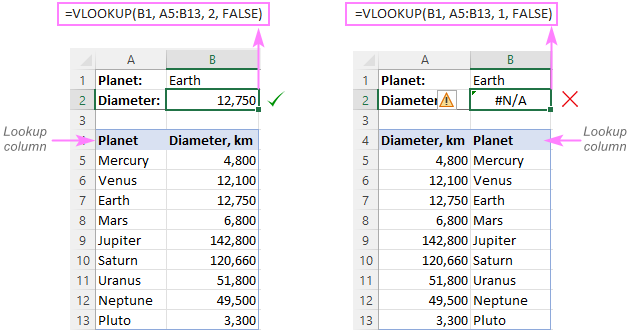Solution: If it is not possible to restructure your data so that the lookup column is the left-most column, you can use the INDEX and MATCH functions together as an alternative to VLOOKUP. Here's a formula example: INDEX MATCH formula to look up values to left.

#### 5. Numbers are formatted as text

Another common source #N/A errors in VLOOKUP formulas is numbers formatted as text, either in the main or lookup table.

This usually occurs when you import data from some external database or if you've typed an apostrophe before a number to show leading zeros.

Here are the most obvious indicators of numbers formatted as text: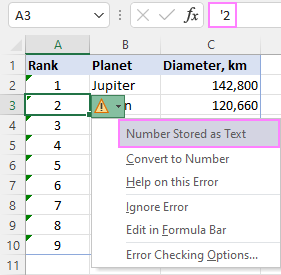Solution: Select all the problematic numbers, click on the error icon and choose Convert to Number from the context menu. For more information, please see How to convert text to number in Excel.

#### 6. Leading or trailing spaces

This is the least obvious cause of the VLOOKUP #N/A error because a human eye can hardly spot those extra spaces, especially when working with big datasets where most of the entries are below the scroll.

Solution 1: Extra spaces in the lookup value

To ensure the correct work of your VLOOKUP formula, wrap the lookup value in the TRIM function:

`=VLOOKUP(TRIM(E1), A2:C10, 2, FALSE)`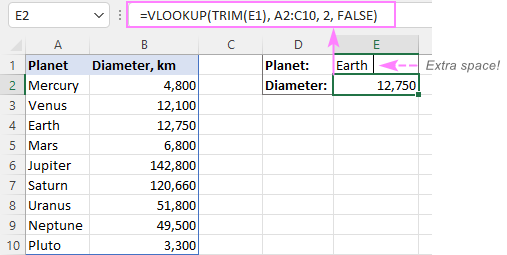Solution 2: Extra spaces in the lookup column

If extra spaces occur in the lookup column, there is no easy way to avoid #N/A errors in VLOOKUP. Instead, you can use a combination of INDEX, MATCH and TRIM functions as an array formula:

`=INDEX(B2:B10, MATCH(TRUE, TRIM(A\$2:A\$10)=TRIM(E1), 0))`

Since this is an array formula, don't forget to press Ctrl + Shift + Enter to properly complete it (in Excel 365 and Excel 2021 where arrays are native, this also works as a regular formula).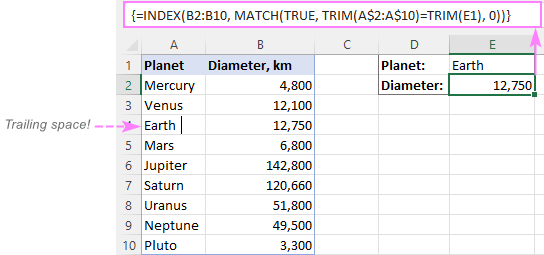Tip. A quick alternative is running the Trim Spaces tool that will eliminate excess spaces both in the lookup and main tables in seconds, making your VLOOKUP formulas error-free.

## #VALUE! error in VLOOKUP formulas

In general, Microsoft Excel displays the #VALUE! error if a value used in the formula is of a wrong data type. In respect to VLOOKUP, there are three common sources of the VALUE! error.

#### 1. Lookup value exceeds 255 characters

Please be aware that VLOOKUP cannot look up values containing more than 255 characters. If your lookup values exceed this limit, a #VALUE! error will be displayed: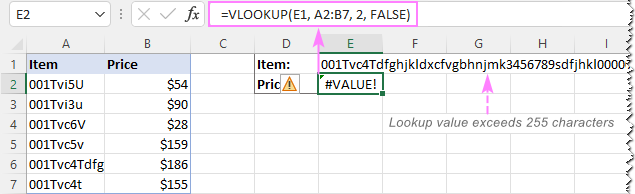Solution: Use an INDEX MATCH formula instead. In our case, this formula works perfectly:

`=INDEX(B2:B7, MATCH(TRUE, INDEX(A2:A7= E1, 0), 0))`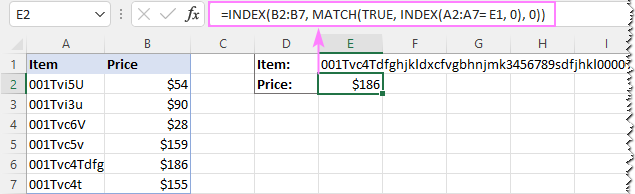#### 2. Full path to the lookup workbook is not supplied

If you are pulling data from another workbook, you have to include the full path to it. More precisely, you have to enclose the workbook's name including the extension in [square brackets] and specify the sheet's name followed by the exclamation mark. If the workbook name or sheet name, or both, contain spaces or any non-alphabetical characters, the path must be enclosed in single quotation marks.

Here's the structure of the table_array argument to Vlookup from another workbook:

`'[workbook name]sheet name'!range`

A real formula might look similar to this:

`=VLOOKUP(\$A\$2,'[New Prices.xls]Sheet1'!\$B:\$D, 3, FALSE)`

The above formula will search for the value of A2 in column B of Sheet1 in the New Prices workbook, and return a matching value from column D.

If any element of the path is missing, your VLOOKUP formula won't work and return the #VALUE error (unless the lookup workbook is currently open).

#### 3. The col_index_num argument is less than 1

It's hard to imagine a situation when someone intentionally enter a number less than 1 to specify the column to return values from. But it may happen if this argument is returned by some other function nested in your VLOOKUP formula.

So, if the col_index_num argument is than 1, your formula will return the #VALUE! error too.

If col_index_num is greater than the number of the columns in the table array, VLOOKUP produces the #REF! error.

## Solving VLOOKUP #NAME error

This is the easiest case - the #NAME? error appears if you've accidentally misspelled the function's name.

The solution is obvious - check the spelling :)

## The main causes of errors in Excel VLOOKUP

Apart from having a fairly complicated syntax, VLOOKUP has arguably more limitations than any other Excel function. Because of these limitations, a seemingly correct formula may often deliver results different from what you expected. Below you will find solutions for a few typical scenarios when VLOOKUP fails.

### VLOOKUP is case-insensitive

The VLOOKUP function does not distinguish the letter case and teats lowercase and uppercase characters as identical.

Solution: Use VLOOKUP, XLOOKUP or INDEX MATCH in combination with the EXACT function that can match text case. You can find the detailed explanations and formula examples in this tutorial: 5 ways to do a case-sensitive Vlookup in Excel.

### A new column was inserted or removed from the table

Regrettably, VLOOKUP formulas stop working every time when a new column is deleted from or added to the lookup table. This happens because the syntax of the VLOOKUP function requires defining the index number of the return column. When a new column is added to/removed from the table array, obviously that index number changes.

Solution: The INDEX MATCH formula comes to the rescue again : ) With INDEX MATCH, you specify the lookup and return ranges separately, so you are free to delete or insert as many columns as you want without worrying about updating every associated formula.

### Cell references change when copying the formula to other cells

The heading gives an exhaustive explanation of the problem, right?

Solution: Always use absolute references (with the \$ sign) for the table_array argument, e.g. \$A\$2:\$C\$100 or \$A:\$C. You can quickly switch between different reference types by pressing the F4 key.

### VLOOKUP returns the first found value

As you already know, Excel VLOOKUP returns the first value it finds. However, you can force it to bring the 2nd, 3rd, 4th or any other occurrence you want. There is also a way to get the last match or all found matches.

Solutions: Formula examples are available here:

## Why does my VLOOKUP work for some cells but not others?

When your VLOOKUP formula returns the correct data I some cells and #N/A errors in others, there can be a few possible reasons why that happens.

1. The table array is not locked

Suppose you have this formula in row 2 (say in E2), which works nicely:

`=VLOOKUP(D2, A2:B10, 2, FALSE)`

When copied to row 3, the formula changes to:

`=VLOOKUP(D3, A3:B11, 2, FALSE)`

Because a relative reference is used for table_array, it changes based on the relative position of the row where the formula is copied, in our case from A2:B10 to A3:B11. So, if the match is in row 2, it won't be found!

Solution: When using a VLOOKUP formula for more than one cell, always lock the table array reference with the \$ sign like \$A\$2:\$B\$10.

2. Text values or data types do not match

Another common reason for VLOOKUP failure is the difference between your lookup value and a similar value in the lookup column. In some cases, the difference is so subtle that it's hard to spot visually.

Solution: When VLOOKUP is returning an #N/A error while you can clearly see the lookup value in the lookup column, and apparently both are spelt exactly the same, the first thing you need to do is to determine the root cause of the problem - the formula or the source data.

To see whether the two values are the same or different, make a direct comparison in this way:

`=E1=A4`

Where E1 is your lookup value and A4 is an identical value in the lookup column.

If the formula returns FALSE, that means the values differ in some way, though they look absolutely alike.

In case of numeric values, the most possible reason is numbers formatted as text.

In case of text values, most likely the problem is in excess spaces. To verify this, find out the total length of the two strings using the LEN function:

`=LEN(E1)`

`=LEN(A4)`

If the resulting numbers are different (like in the screenshot below), then you've pinpointed the culprit - extra spaces: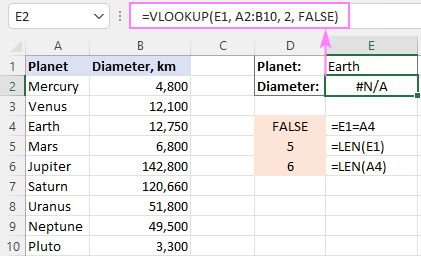To solve the issue, either remove extra spaces or use this INDEX MATCH TRIM formula as a workaround.

## Why does my VLOOKUP pull wrong data?

There could be even more reasons why your VLOOKUP returns a wrong value:

1. Incorrect search mode. If you want an exact match, be sure to set the range_lookup argument to FALSE. The default is TRUE, so if you omit this argument, VLOOKUP will assume you are looking for an approximate match and search for the closest value that is smaller than the lookup value.
2. The lookup column is not sorted. For approximate match VLOOKUP (range_lookup set to TRUE) to work correctly, the first column in the table array must be sorted in ascending order, from smallest to largest.
3. Duplicates in the lookup column. If the lookup column contains two or more duplicate values, VLOOKUP will return the first found match, which may not be the one you expect.
4. Incorrect return column. Double-check the index number in the 3rd argument :)

## VLOOKUP not working between two sheets

First off, it should be noted that the common reasons of #N/A, #VALUE, and #REF errors discussed above may cause the same problems when looking up from another sheet. If it's not the case, check out the following points:

1. Make sure the external reference to another sheet or a different workbook is correct.
2. When doing a Vlookup from another workbook which is closed at the moment, verify that your formula contains the full path to the closed workbook.
3. If VLOOKUP cannot pick a table array in another worksheet (i.e. when you highlight a range in the lookup sheet, nothing appears in the table_array argument in the formula or in the corresponding box of the formula wizard), then most likely the two sheets are open in separate instances of Excel and cannot communicate with each other. For more info, please see How to determine which Excel files are in which instance. To fix this, simply close all Excel windows, and then reopen the sheets/workbooks in the same instance (the default behavior).

## How to Vlookup without errors in Excel

If you do not want to intimidate your users with standard Excel error notations, you can display your own user-friendly text instead or return a blank cell if nothing is found. This can be done by using VLOOKUP with IFERROR or IFNA function.

### Catch all errors

In Excel 2007 and later, you can use the IFERROR function to check a VLOOKUP formula for errors and return your own text (or an empty string) if any error is detected.

For example:

`=IFERROR(VLOOKUP(E1, A2:B10, 2, FALSE), "Oops, something went wrong")`

In Excel 2003 and earlier, you can use the IF ISERROR formula for the same purpose:

`=IF(ISERROR(VLOOKUP(E1, A2:B10, 2, FALSE)), "Oops, something went wrong", VLOOKUP(E1, A2:B10, 2, FALSE))`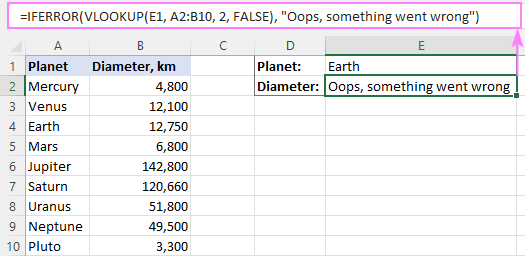For more details, please see Using IFERROR with VLOOKUP in Excel.

### Handle #N/A errors

To trap only #N/A errors ignoring all other error types, use the IFNA function (in Excel 2013 and higher) or IF ISNA formula (in all versions).

For example:

`=IFNA(VLOOKUP(E1, A2:B10, 2, FALSE), "Oops, no match is found. Please try again!")`

`=IF(ISNA(VLOOKUP(E1, A2:B10, 2, FALSE)), "Oops, no match is found. Please try again!", VLOOKUP(E1, A2:B10, 2, FALSE))`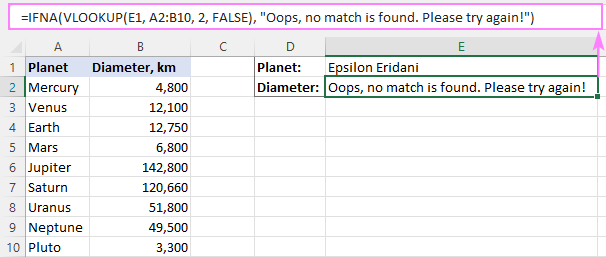That's all for today. Hopefully, this tutorial will help you get rid of VLOOKUP errors and have your formulas working in the way you want.

## You may also be interested in

1. Hi Sir
Would you please help to sort out my problem? I have a work sheet where VLOOKUP working properly but when i add a cell value that contain sheet name using formula =MID(CELL("filename",A1),FIND("]",CELL("filename",A1))+1,255). No issue at all, adding sheet name manually in that cell. but adding with formula i got error.

2. I have copied a Vlookup down a number of rows in sections of a worksheet but occasionally the relative cell reference being checked against the other data source, for e.g. D3 to fill data in B3 and C3 changes to D5 although is in a protected cell and always seems to jump by 2 rows? Would you have any ideas please? Many thanks.

• Hi!
If you use a relative cell reference and copy the formula down the column, only the row number may change. The column cannot change. Therefore, I cannot understand your problem.
Write what formula you use and what actions you perform.

• Hi,

Thanks for your help on this and sorry for not being clearer.

I have formula below in AM68:
=IFERROR(VLOOKUP(AP68,NEAlloc!\$A\$4:\$U\$300,5,FALSE),"")

and this one in AN68:
=IFERROR(VLOOKUP(AP68,NEAlloc!\$A\$4:\$U\$300,7,FALSE),"")

I have then copied this down a number of rows underneath and generally works okay but sometimes the row number changes even though it’s in a locked protected cell. So for example AP68 might become AP70 in both AM68 and AN68. I have a number of blocks on same worksheet set up in this way.

I hope you can see the situation now.

I look forward to hearing back from you.

Many thanks

Julian

• Hi!
When you copy a formula from AM68 to AM69, the relative reference in the formula should change. You will see in AM69 -

=IFERROR(VLOOKUP(AP69,NEAlloc!\$A\$4:\$U\$300,5,FALSE),””)

This relative reference will also change if you insert or delete rows above the formula.

• put coma after the column and wrire FALSE and close the bracket
for example \$C\$8:\$l\$120,2,FALSE

3. Hi,
I need help as i use Vlookup formula to identify the value and return the value
example
L122279 School ED which divide in 3 column.
however when i used the vlookup in table it unable to identify the number and return the value NA.

• Hi!
What formula are you using and what value are you looking for?

4. how to use Vlookup with If AND or function

5. Hi there,

I am attempting to create a sheet in my workbook designated to 'lookup' specific pricing for two seperate inputs (Customer & ZipCode). I have 20 different 'Customer' sheets & the VLOOKUP format I'm using has worked on 11 of them without flaw. The other 9 give me the #N/A error. One of these sheets I manually went in and simply double clicked every cell in the 'look-up column' and mysteriously solved the error for that specific sheet.

The remaining sheets I cannot figure out why I am still getting the #N/A error. I've tried everything from changing formats, checking for excess spaces, double clicking into each cell & even deleting the entire column and retyping 200+ zip codes into a new column (this was painful when it didn't work).

I have searched Hi & Low for a solution but nothing I have come across matches my issue. If you have any ideas I'm open to try anything at this point! I find it very odd that half the sheets work fine, the other half do not.

Thank you

• Hello!
Unfortunately, without seeing your data it is difficult to give you any advice. Perhaps your zip code is written as text.
You can send us a small sample workbook with the source data and expected result to support@ablebits.com. Please shorten your tables to 10-20 rows/columns and include the link to your blog comment.

6. Hi
Whenever i perform a function, say IF. The formula doesn't wanna give the results. It pops a message saying There's a problem with this formula. But when i use the very same IF function on a different Excel (Laptop), its giving me the complete results.

I even retrieved my old work where I applied the functions and accessed the cell then clicked enter to check it, it now says "there's a problem with this formula"

what would be the reason, Is there something i can do with the formula to work perfectly?

7. When using vlookup formula. It's as if it's just typing in the box. Not actually processing the formula. Does anyone why why this is?

• Hi,
I’m sorry but your task is not entirely clear to me. For me to be able to help you better, please describe your task in more detail. Please specify what you were trying to find, what formula you used and what problem or error occurred. Give an example of the source data and the expected result.
It’ll help me understand it better and find a solution for you.

8. Thank you! I couldn't figure out why I kept getting that darn N/A error message. Your tip about putting the lookup column as the first column on my lookup table resolved my issue!

9. Hi
I am doing Index and Match it does no work in the whole column and appear as N/A. The message comes up that the column is not synchronised.
Would anyone help how can I solve the problem?
My Excel is 2007.

Thanks

• Hello!
If an INDEX MATCH formula in Excel cannot find a lookup value, it produces an #N/A error.
Unfortunately, without seeing your data it is difficult to give you any advice. Please provide me with an example of the source data and the expected result. Please specify what you were trying to find, what formula you used. It’ll help me understand it better and find a solution for you.

10. hi Alexander,

You were right.
The trim and cleam formula =trim(clean(a2)) works very well

THANK YOU!

11. hi

I am using Vlookup on a very long list of "itemnumbers."
These "itemnumbers" consist of a combination of letters and numbers.
Some consist of numbers only.
Some consist of letters only.

All columns were set as text, prior to pasting the values.
Very often, Vlookup does not correctly find all of the "itemnumbers" which consist of numbers only.

0000123456789 vlookup does not find this
000123ABC0012 vlookup does find this
ABCDEABCDEXYZ vlookup does find this
ABC7890123456 vlookup does find this
8888885521111 vlookup does not find this

To fix this, i can go into each cell [F2] then hit [ENTER]
After that, vlookup does find a match.

Does anybody know of a better way to do this?

---
By the way,
The "extra spaces" problem, I usually "solve" by CTR+H … then replace empty spaces with nothing
That obviously works only when the data is not supposed to have any empty spaces
--

• Hello!
I could not repeat your mistakes. Your data may have come from another program. The data may contain extra spaces and non-printing characters. You can learn more about deleting spaces and non-printing characters in Excel in this article on our blog.

• hello Alexander

Thank you for trying.

yes, the data comes from different sources
1 huge list of itenmumbers from an ERP (with all sorts of info in other columns)
1 huge list of itemnumbers from another source (with different info in other columns)
I then use vlookup in excel to combine the data in 1 sheet.

To be able to do so, first i get all data to .dat or .txt to remove formats
Then I set a new empty excel sheet to text completely (not to general / not to number) this is very important.
Then I copy/paste values only to excel.

If there are any empty spaces, these are deleted with the CTR+H (find space and replace with nothing, works perfectly.)

To me, the resulting values from both sources look identical.
Excel does correctly match all of the text fields which contain a combination of letters and numbers (even if the first character is a zero 0)
Excel does correctly match all of the text fields which contain letters only.

However, excel does not correctly match the textfields which consist of numbers only.
By chance I found a labour intensive workaround
(after hitting ENTER while reviewing the data in a cell which should have been found by Vlookup.. suddenly the Vlookup did work for that 1 cell)

...so... I hit [F2] [ENTER] many, many times... and then it does work.

I was hoping somebody would know of a more efficient way.
Maybe there is some trick to mimic [F2] [ENTER] in all cells of a column.

• Hello!
Your data contains some kind of non-printable characters. I recommend using the article, the link to which I gave you earlier. I think this will help.

12. I applied vlookup from a sheet...the sum of result which I got through vlookup is greater than the sum in the original file ...how is this possible?

13. IN MY WORKSHEET BY MISTAKE I PRESSED SOME KEYS. IT SHOWS AS #VALUE! ... I TRIED IN MANY WAYS . BUT I COULDN'T GET IT AGAIN. ALL THE TEXT ENTRIES ONLY CHANGED AS #VALUE!. IN A WORKBOOK ALL THE 4 SHEET GOT THE SAME PROBLEM. GUIDE ME HOW TO SOLVE IT?

• Hello!

14. Hi there,

Can someone help me with this formula for vlookup?

=VLOOKUP(P\$1:P\$32424,'Report 341.05'!A1:AM56914,17,FALSE) is not bringing me any data in the spreadsheet.

Thanks!

• Hello!
Without seeing your data it is difficult to give you any advice.
But Lookup_value cannot be a range (P\$1:P\$32424). Lookup_value can be a value (number, date or text), cell reference (reference to a cell containing a lookup value), or the value returned by some other function.

15. Does anyone know why when typing a vlookup statement, the cell displays something like this =vlookup(A2,Table_Name,8,false). excel does not run the statement? just displays the characters of the statement. TIA

16. So my current issue is number with decimals. I got several sets of data with 123456.xyz. XYZ changes on each of them. I tried to vlookup with first 6 numbers, it wont detect if there is decimals involved. Any work around for that?

• Thanks for the reply, tho in my case number after decimals important for me so rounding doesn't help. I was basically looking a way to sort the numbers based on values before decimals as they are fixed and values after decimals change. I guess VLOOKUP function can't help with that. Back to the drawing board!

• Hello!
You can round to 2 or 3 decimal places that are important to you.

17. ANOTHER POSSIBLE REASON VLOOKUP GET #N/A

If the lookup column has multiples of the same value it confuses lookup and will cause the #/NA error. In other words, the lookup value must have a unique presence in the lookup column.

• Hi Jason,

In case of multiple matches, VLOOKUP returns the first found match. This may be confusing in many situations, so I agree that you'd better have unique values in the lookup column.

18. I am having a format issue with vlookup.
If I copy from the vlookup data sheet like an id number it will work but if I type the id number it will return #NA.
- both data and input sheet have the column set as numbers
-tried copying and pasting the formatting from data to input sheet
Any help is appreciated :)

• Hello!
If your numbers are derived from calculations, I recommend using rounding. Your numbers may differ in decimal place, which is not shown on the screen.

19. one cell is having more than 100 values. so vlookup failed and getting NA but if use find it finding the value.

• Hello!
Clarify the question. One cell can only have 1 value

20. Hi. Really appreciate the write up. I am using excel for Mac. I used vlookup to match serial numbers. For the serial numbers, I have to use the tick mark ' to keep leading 0s. Vlookup is not matching not matter what I have tried. The odd thing is that if I double click in the first cell in the array table, the tick mark is removed and the match occurs. I am trying to avoid double clicking on thousands of cells to make vlookup work. Any ideas? Kind of urgent for a data conversion. THANKS!

• yeah me experience this weird error too. please any tips ASAP.

21. Thank you so much. Really appreciate it.

22. The above guide still not solve my problem. I still
Hitting NA# in the VLOOKUP after I done edit or amend the cell format. Can I send u the file ? And you help to point out the issue ?

• Hello Victoria!
I’m sorry but your task is not entirely clear to me. For me to be able to help you better, please describe your task in more detail. Please specify what you were trying to find, what formula you used and what problem or error occurred. Give an example of the source data and the expected result.
It’ll help me understand it better and find a solution for you. Thank you.

23. I have a simple VLOOKUP table with unordered data. I have set up the formula properly (set to exact match, to lookup value in left col and return value in the right). Format is general for both the array and for the col of values being looked up (I don't have, for example, numbers with the apostrophe in front, indicating that they are text. The formula works for all values, except as follows:
ANY VALUE THAT CONTAINS PARENTHESES RETURNS AN #N/A, EVEN THOUGH THE IDENTICAL VALUE IS IN THE LEFT COL OF THE ARRAY. I have checked character counts, I have used EXACT in a sample case to confirm that the values in the array and in the col of values that I am looking up are identical—there are no extra spaces. I have deleted the parens and confirmed that I get a value, not an #N/A. I have replaced the parens with slashes—the formula works. I HAVE REPLACED THE PARENS WITH BRACKETS—THE FORMULA RETURNS AN #N/A.
Is this a known bug?

• Hello!
It’ll help me understand it better and find a solution for you. Thank you.

• Thanks, Alexander! It's real simple—I am trying to confirm that a value in one col of my doc appears verbatim in another. Here is the set up:
Col A
value 1, 2, 3, etc. [different value in each row—the values are not ordered]
Col B
OK [I use the value "OK" in each row]
Col C
value 1, 2, 3, etc. [different value in each row—there cannot be a value in C that does not appear in A]
Col D
=VLOOKUP(C4;\$A\$1:\$B\$75;2;0) [this is the formula in row 4 of the doc—the first cell reference, Cx, always matches the given row]
I want to this formula in D to give me an #N/A if the value in C is not found in A, but an "OK" if the value in C appears in A. Very simple.
However, what I discovered is that, as soon as there are parentheses in the value, it doesn't matter if the value in C appears in A exactly as it appears in C—I get an #N/A. When I delete the parens in both A and C, I get my OK instead of the #N/A.

I am familiar with the problems created for EXACT, vLOOKUP, and other formulas by trailing space, by numbers converted to text, and so on. I am pretty confident that my problem is not due to these issues. Instead, I think the appearance of parentheses or brackets in the text causes vLOOKUP to fail. I was hoping folk could confirm—and perhaps supply a workaround. (Yes, I can replace the parens with another character before using vLOOKUP. Might there be something else to do in the formula?)

Thanks for any light you can shed!

• Hello!
The problem with parentheses in the VLOOKUP function is unknown to me. This did not cause problems for me. Maybe after the bracket there is still a space or some non-printable character? Try using the TRIM or CLEAR function:

= VLOOKUP(CLEAR(TRIM(C4));\$A\$1:\$B\$75;2;0)

I hope this will help, otherwise please do not hesitate to contact me anytime.

24. My formula was VLOOKUP(C2,Sheet2!A2:B45,2,FALSE). The expectation was that the output should be the value from column B on Sheet2 if there is a match. However, it never returned a value. Any ideas?
A similar VLOOKUP worked on the same sheet with no issues.

• Hello!
Your VLOOKUP formula has no errors. I don’t know what values you are looking for, but I suppose it is text. Perhaps there are extra spaces, non-printable characters in the text. I recommend checking for extra spaces and non-printing characters, as described in this article.

25. Hi ,
While using Vlookup ,
Lookup value is a formula based reference created using the formula
"=TRIM(LEFT(A4,5)&MID(A4,10,3)&RIGHT(A4,4))" . A4 cell value is = 10211-40-000-0000.
after allpying the above formula i get value as "102110000000" in B4.
Now if i put vlookup or index match taking "B4 cell" as lookupvalue i m getting error as "#N/A" . However if paste special the same cell with values the formula works fine .
Regards
Praveen L

26. That has really fixed my problem after long time searching, Thank you !!

27. The numbers formatted as text gets me every time

• Hello Pat!
Please describe your problem in more detail. It’ll help me understand it better and find a solution for you. Thank you.

28. Hi
How do I get the 0 to return as text in my formula, this leads on to pivot table and if it can find the value it presents as text but if it cant it presents as value so I get two lines in my pivot? =IFERROR(VLOOKUP(A2,'[Works in RAMM by month 19-20.xlsx]Sept to June 20'!\$A\$1:\$L\$276,12,FALSE),"0 ")
thanks Mo

• Hello Maureen!
If I understand your task correctly, maybe the following formula should work for you:

=IFERROR(VLOOKUP(A2,'[Works in RAMM by month 19-20.xlsx]Sept to June 20'!\$A\$1:\$L\$276,12,FALSE),"0" & " ")
Hope you’ll find this information helpful.

29. i am having a lookup related problem when i am applying lookup function to a cell which does not contain the actual data but there is formula applied to get the value from other two cell so now lookup is unable to look for that cell where formula has been filled but when i am typing actual value in that cell lookup function is working correctly but when there is a formula which is showing correct value lookup function is unable to work .
For example lookup can look for a value 3 and search it in an array but when there is two column containing 1 in first column and 2 in second column and now in third column i am using formula to add them simply so i sum them and now the value i am getting is 3 in third column but when i am applying lookup function to it. It is showing #N/A as usual but when instead of formula i am directly typing the value 3 in third column the lookup formula works perfectly so any solution on this will be helpful.

• Hello Rajat!
Most importantly, what function are you using for a search? Is it VLOOKUP or anything else? Please give me the formula which is performing a search.
What data are in the cells which that formula is referring to?

• Hello Alexander,

I have a similar problem like Rajat. The 'lookup_value' reference in my Vlookup function is actually a cell with formula which is =RIGHT(A1,3). But vlookup is unable to read this and returning #N/A error, if I manually put the result of =RIGHT(A1,3) then vlookup is able to return the result. Also please note that if the result of the function =RIGHT(A1,3) is text then it work but unfortunately my result for this function is a number, so I guess this has something to do with formatting of the cell or something.

GK

• Hello!
Calculation result RIGHT(A1,3) - always text. If you need to search for a number, then I recommend using = VALUE(RIGHT(A1,3))

• Hi

Working perfectly!!! Thank you so much!!!

GK

• I had the same problem,

Thanks

30. hello,

can you help me_ please. This function =IFERROR(SVYHLEDAT(A3;'M (3)'!A3:I9988;3;1);"Not")
do not describe. Why?

Thanks

31. VLOOKUP() will fail if, "the lookup column is not sorted in the ascending order." Well, that's just stupid.

33. When using vlookup, I can get the correct results for most of the table. When I get down to the last 4 lines of the table, it gives me results from previous lines when i change the lookup data. I have resorted the table multiple times but always get the same incorrect response.

34. I am using vlookup to copy values from one table to another. Many of the values have been copied, but not all. Those that have not been copied are showing #NV. I have checked for all the possible reasons for the error, corrected some, but the problem persists and has not changed at all. I even tried INDEX/MATCH with no avail. Any Idea what could be the cause?

35. Thank you for this, very helpful!

36. Thanks for this outstanding reference, much appreciated! Also, good to know I'm not the only one that struggles with this in Excel.

37. When using vlookup, the formula only displays moot the value. No error messages. Any ideas?

38. Hi, i use vlookup to match in the completion status from another file. but then some of my cell does not capture the formula and turn out the result is wrong. would anyone please help me out in this matter.

39. i am using vlookup from another sheet, i have entered 3 entries that is ok but when i am doing next entry it pulls value from wrong row. please help me i have my file if you ask me i can mail you my file.

40. Another possible issue: special characters in lookup value. E.g. tilde ~. Solution would be to duplicate this character: VLOOKUP(SUBSTITUTE(C1,"~","~~"),A:B,2,false)

41. excellent ,thank you

42. Trying to do a vlookup on a table that is 1000 rows and 26 columns. If I try to get the value in the 13th column, it works fine. However, if I try for any column greater than that I get a #REF! error. All columns have valid data. Formula is =VLOOKUP(\$a1,TableSheet!\$A:\$Z,15,FALSE). Any ideas?

• If you want to get work done, and run into excel misbehaving, go find an old copy of Lotus 1-2-3, install it, copy your data in and do your lookups there. The Lotus vlookup function isn't nearly as stupid or sensitive as excel, and doesn't require the lookup table data to be sorted just so to work properly.

43. I have data in two different excel files. I tried to input VLOOKUP manually.

44. =VLOOKUP(IF(BC10>=130%,"130% & Above",IF(BC10<70%,"0.0 - 69%",ROUNDDOWN(BC10,2))),INDIRECT(BL10),2,0)
so the result should show rating
Sales Target RM25mil & Above
Rating Rating Range Ach % Rating Ach %
Outstanding 4.75-5.00 126% - Above 5.00 130% & Above
4.94 129%
4.88 128%
4.81 127%
4.75 126%
4.50-4.74 121% - 125% 4.74 125%
4.68 124%
4.62 123%
4.56 122%
4.50 121%

45. Very Useful - keep up the good work ( share the knowledge..sharing is caring )

46. THANK YOU. THIS IS QUITE USEFUL.

47. The iferror function work greatly, the if(iserror way may still produce #VALUE! cells.

48. I did a vlookup and the output showed this number (15359E+11) instead of the actual digits. I have not had this kind of error before. Please advise.

• Just convert it to NUMBER

49. Hi,

I have problem with vlookup, if i perform the vlookup in the same file with different sheets, the formula is giving me partial results. However if i vlookup the same data from a different excel file, its giving me the complete results.

what would be the reason, Is there something i can do with the formula to work perfectly within sheets?

50. =IF(K9="one",IFERROR(VLOOKUP(N9,[One.xlsx]Ledger!\$A\$12:\$BI\$29,3,FALSE),"No Data"))&IF(K9="two",IFERROR(VLOOKUP(N9,[Two.xlsx]Ledger!\$A\$12:\$BI\$29,3,FALSE),"No Data"))&IF(K9="three",IFERROR(VLOOKUP(N9,[three.xlsx]Ledger!\$A\$12:\$BI\$29,3,FALSE),"No Data"))

Result FALSEMANISHA BISTAFALSE

how to remove FALSE